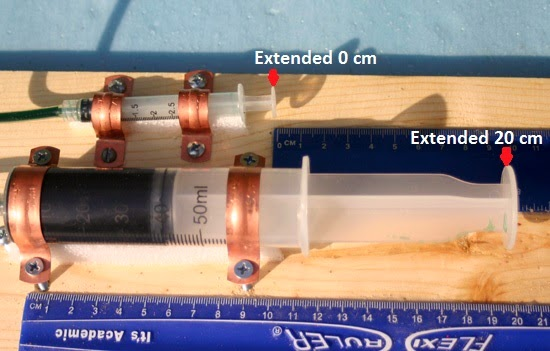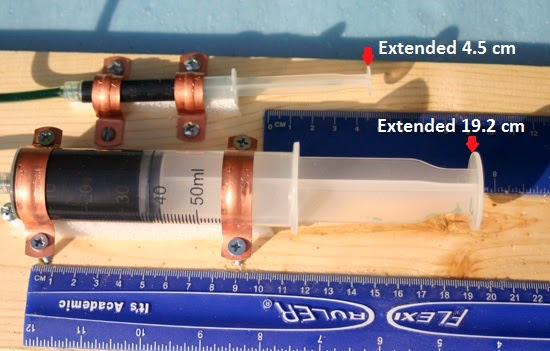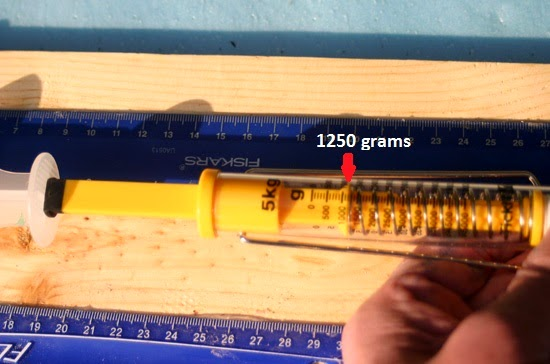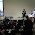## Monday, March 31, 2014

### Proving Pascal’s Principle With Syringe Hydraulics

The Syringe Hydraulics Arm project on my website has been one of the most popular project articles. Lately I have been working on building a simulator to demonstrate Pascal’s Principle of fluids using syringes and plastic tubing.

“Pascal's Principle states that when there is an increase in pressure at any point in a confined fluid, there is an equal increase at every other point in the container.”

What exactly does this mean in practice?  For the simulator I used a large syringe that has a piston cross section diameter of 34 mm and small syringe with cross section diameter of 13 mm.  Like other mechanical systems there is a mechanical advantage where distance moved and force tradeoff. When the smaller piston is pushed with a force, that force is distributed equally across the larger piston cross section causing a greater net force.   For the fluid to be spread across the larger cross section more fluid volume must be moved from the smaller cylinder.

For my first experiment I worked from the other direction and pushed the large cylinder a short distance of 8 mm which extended the small cylinder a much longer distance of around 45 mm until it could not move any farther. I calculated this also which was off somewhat from my observations which can happen when there are inaccuracies in the measured values.Cylinder at Start PositionLarge Cylinder Moved 8 mm Small Cylinder Moved 45 mm

For calculations we need the formula for the area of a circle :

Area of Circle = π x radius²
Large Piston   cross section area = 3.14 x (34/2)²  =   907 sq mm
Small Piston  cross section area = 3.14 x (13/2)² =  133 sq mm

Moving the large piston 8 mm will displace amount fluid = cross section x length of movement

Fluid Displaced = 907 sq mm x 8 mm = 7256 cu mm
The movement of the small cylinder should be the fluid displaced / cross section area of small cylinder.
7256 / 133 = 54 mm   movement of small cylinder
Actual movement was recorded at 45 mm or 4.5 centimeters

I have not checked the amount of force generated but did check the amount required just to move the opposite cylinder.  Moving the small cylinder with the large cylinder took a large amount of force, 1250 grams or around 12 newtons. This is like pushing down on the short end of a lever.  Pushing the small cylinder took very little force.Large Force Needed to Move Small Cylinder Pushing Large CylinderSmall Amount of Force to Move Pushing Small Cylinder

Bill Kuhl

1.2.3.